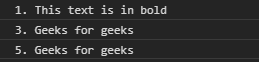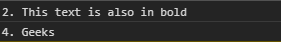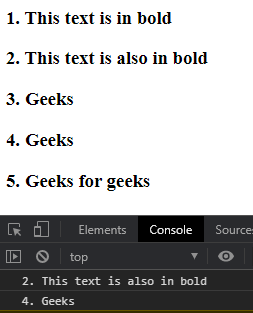# D3.js selection.filter() Function

The d3.selection.filter() function in d3.js is used to filter the given selection and return a new selection for which the filter is true. The filter to be used may be a string or a function.

Syntax:

`selection.filter(filter);`

Parameters: This function accepts one parameter as mentioned above and described below:

• filter: It is a string or a function that would be used to filter a selection. The filter is applied to each selected element when using a function.

Return Values: This function returns the new selection.

Example 1: This example selects all the odd children of the specified element.

## HTML

 ` ` `<``html` `lang``=``"en"``> ` ` `  `<``head``> ` `    ``<``meta` `charset``=``"UTF-8"``> ` `    ``<``meta` `name``=``"viewport"` `content``= ` `        ``"width=device-width,initial-scale=1.0"``> ` `     `  `    ``<``script` `src``= ` `        ``"https://d3js.org/d3.v4.min.js"``> ` `    `` ` `    ``<``script` `src``= ` `        ``"https://d3js.org/d3-selection.v1.min.js"``> ` `    `` ` ` ` ` `  `<``body``> ` `    ``<``div``> ` `        ``<``b``>1. This text is in bold ` `        ``<``b``>2. This text is also in bold ` `        ``<``b``>3. Geeks for geeks ` `        ``<``b``>4. Geeks for geeks ` `        ``<``b``>5. Geeks for geeks ` `    `` ` `    `  `    ``<``script``> ` `        ``let selection = d3.selectAll("b") ` `            ``.filter(":nth-child(odd)") ` `            ``.nodes(); ` `        ``selection.forEach((e) => { ` `            ``console.log(e.textContent) ` `        ``}) ` `    `` ` ` ` ` `  ` `

Output:Example 2: This example selects all the even children of the specified element.

## HTML

 ` ` `<``html` `lang``=``"en"``> ` ` `  `<``head``> ` `    ``<``meta` `charset``=``"UTF-8"``> ` `    ``<``meta` `name``=``"viewport"` `content``= ` `        ``"width=device-width,initial-scale=1.0"``> ` `    ``<``script` `src``= ` `        ``"https://d3js.org/d3.v4.min.js"``> ` `    `` ` `    ``<``script` `src``= ` `        ``"https://d3js.org/d3-selection.v1.min.js"``> ` `    `` ` ` ` ` `  `<``body``> ` `    ``<``div``> ` `        ``<``b``>1. This text is in bold ` `        ``<``b``>2. This text is also in bold ` `        ``<``b``>3. Geeks ` `        ``<``b``>4. Geeks ` `        ``<``b``>5. Geeks for geeks ` `    `` ` `     `  `    ``<``script``> ` `        ``let selection = d3.selectAll("b") ` `            ``.filter(":nth-child(even)") ` `            ``.nodes(); ` `        ``selection.forEach((e) => { ` `            ``console.log(e.textContent) ` `        ``}) ` `    `` ` ` ` ` `  ` `

Output:Example 3: This example uses selection.selectAll as a filter.

## HTML

 ` ` `<``html``> ` ` `  `<``head``> ` `    ``<``meta` `charset``=``"UTF-8"``> ` `    ``<``meta` `name``=``"viewport"` `content``= ` `        ``"width=device-width, initial-scale=1.0"``> ` ` `  `    ``<``script` `src``= ` `        ``"https://d3js.org/d3.v4.min.js"``> ` `    `` ` `    ``<``script` `src``= ` `        ``"https://d3js.org/d3-selection.v1.min.js"``> ` `    `` ` ` ` ` `  `<``body``> ` `    ``<``div``> ` `        ``<``h3``>1. This text is in bold ` `        ``<``h3``>2. This text is also in bold ` `        ``<``h3``>3. Geeks ` `        ``<``h3``>4. Geeks ` `        ``<``h3``>5. Geeks for geeks ` `    `` ` `     `  `    ``<``script``> ` ` `  `        ``// Using selection.selectAll with filter ` `        ``let selection = d3.selectAll("div") ` `            ``.selectAll("h3") ` `            ``.filter(":nth-child(even)") ` `            ``.nodes(); ` `        ``selection.forEach((e) => { ` `            ``console.log(e.textContent) ` `        ``}) ` `    `` ` ` ` ` `  ` `

Output:Whether you're preparing for your first job interview or aiming to upskill in this ever-evolving tech landscape, GeeksforGeeks Courses are your key to success. We provide top-quality content at affordable prices, all geared towards accelerating your growth in a time-bound manner. Join the millions we've already empowered, and we're here to do the same for you. Don't miss out - check it out now!

Previous
Next